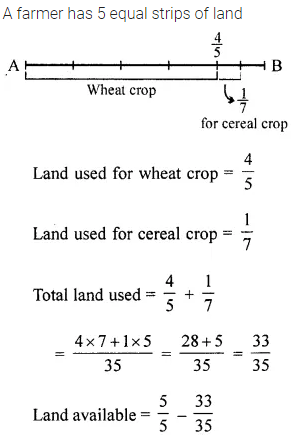# ML Aggarwal Class 6 Solutions for ICSE Maths Chapter 6 Fractions Check Your Progress

## ML Aggarwal Class 6 Solutions for ICSE Maths Chapter 6 Fractions Check Your Progress

Question 1.
State whether the following statements are true (T) or false (F):
(i) The fraction $$\frac{2}{3}$$ lies between 2 and 3.
(ii) To find an equivalent fraction to a given fraction, we may add or subtract the same (non-zero) number to its numerator and denominator.
(iii) To add or subtract like fractions, we add or subtract the numerators while keeping the denominator same.
Solution:Question 2.
How many natural numbers are there between 102 and 112? What fraction of them are prime numbers?
Solution: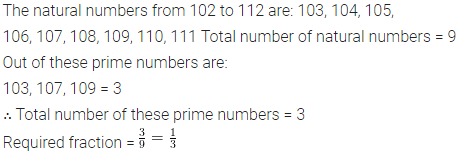Question 3.
Match the equivalent fractions from each row: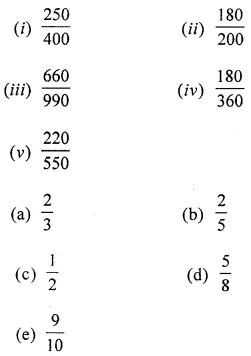Solution: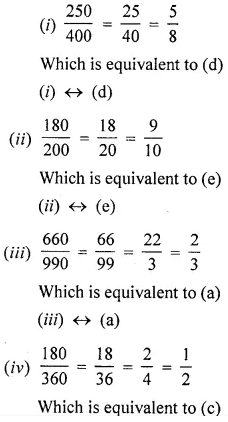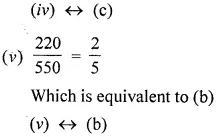Question 4.
Replace by an appropriate symbol ‘< or >’ between the given fractions: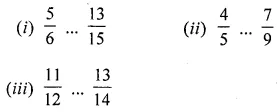Solution: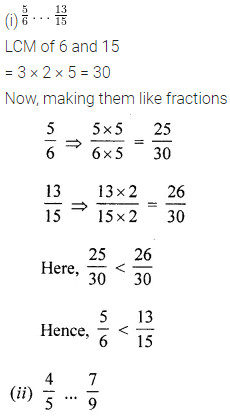Question 5.
Arrange the following fractions in descending order : $$\frac{7}{30}, \frac{13}{15}, \frac{9}{10}, \frac{3}{5}$$
Solution: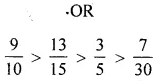Question 6.
Simplify: $$2 \frac{1}{2}-3 \frac{1}{4}+5 \frac{5}{6}$$
Solution: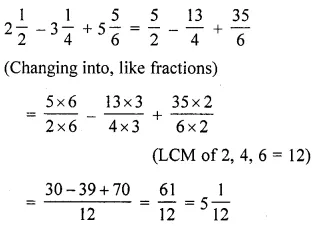Question 7.
Evaluate the following:Solution: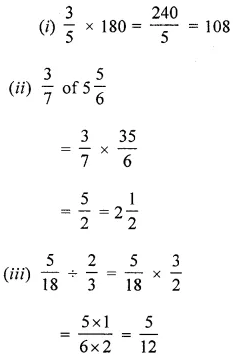Question 8.
Asha and Samuel have bookshelves of the same size partly filled with books. Asha’s shelf is $$\frac{5}{6}$$ full and Samuel’s shelf is $$\frac{3}{5}$$ full. Whose bookshelf is more full and by what fraction?
Solution: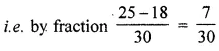Question 9.
A farmer uses four out of five equal strips of his land for wheat crop and $$\frac{1}{7}$$ of his land for cereal crop. What fraction of his land is available for other crops?
Solution: